Latest SSC jobs   »   Area of Triangle

# Area of Triangle: Formulas With Examples

Area of Triangle: Formulas With Examples are provided in this post. A triangle is a polygon, a 2-dimensional object with 3 sides and 3 vertexes. The area of triangular shapes is determined by using a simple formula to be used while solving problems or questions. You must know the length of sides, the type of triangle, the height of the triangle in order to find the area of a triangle. What is the formula to determine the area of Triangles? This post will answer all your queries related to the area of triangles.

Get free notes of Maths

### Area of Triangle: Definition & Types of Triangle

A triangle is a two-dimensional polygon having 3 sides and 3 angles. An area of a triangle is the region occupied inside the triangle. A triangle can be of 4 types depending upon the length of its sides or angles. The 4 types of triangle are:

1. Right Angled Triangle- In which one angle is of 90 degrees.
2. Isosceles Triangle- In which 2 sides are equal.
3. Equilateral Triangle- In which all the three sides are equal and hence each angle is of 60°
4. Scalene triangle- In which all the three sides are unequal.

### Area of Triangle Formula

The general formula for the area of triangle is given by 1/2 x Base x Perpendicular. The area will depend upon the type of triangle. Let’s take a look at the formula to find the area for each type of triangle.

### Area of Right Angled Triangle Formula

A right-angled triangle is a triangle in which one angle is of 90°. The relation between the sides and angles of a right-angled triangle is the basis for trigonometry. The side opposite to the right angle is the hypotenuse. The formula to determine the area of the right-angled triangle is given below.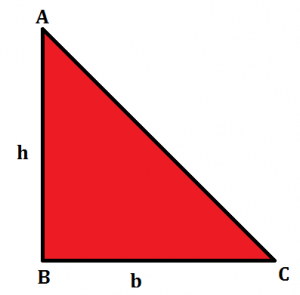In the right-angled triangle given, we have the perpendicular as ‘h’ and base as ‘b’ So the formula for the area of a right-angled triangle can be given by:

### Area of Isosceles Triangle Formula

In an isosceles triangle, you will have 2 sides of equal lengths. Hence, 2 angles of this triangle will be equal to one another. In the figure given below, we have an isosceles triangle with two equal sides, ‘a’ and the base as ‘b’. A perpendicular is drawn from A to D which divides the base into 2 equal parts.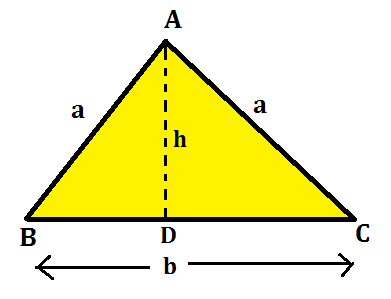Hence, by the formula, A = 1/2 x b x h, we can derive the formula for the area of the isosceles triangle by the formula given below:

### Area of Equilateral Triangle

In an equilateral triangle, you will have the 3 sides of equal lengths. Hence, all the 3 angles of this triangle will be equal and of 60 degrees. In the figure given below, we have an equilateral triangle with equal sides as ‘a’. A perpendicular is drawn from A to D which divides the base into 2 equal parts.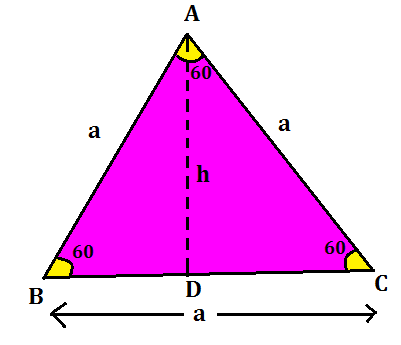Hence, by the formula, A = 1/2 x b x h, the formula for the area of the equilateral triangle can be derived as:

### Area of a triangle by Heron’s Formula

A scalene triangle is a triangle in which all the 3 sides are unequal and no angle is of 90 degrees. To find the area of a scalene triangle and even other triangles, we use the Heron’s Formula. The formula given by Heron about the area of a triangle is also known as Hero’s formula.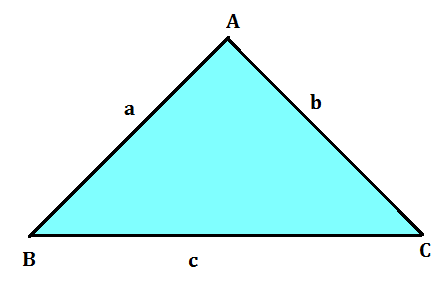From the figure given above, a scalene triangle is given with 3 sides as ‘a’, ‘b’ and ‘c’. The Heron’s formula is stated as: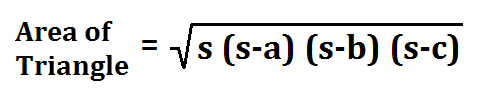where a, b and c are the sides of the triangle, and s = semi-perimeter, i.e., half the perimeter of the triangle. The semi-perimeter of the triangle is given by: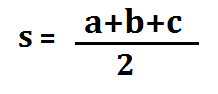### Area of Triangle: Examples

Example 1: Find the area of a triangle, two sides of which are 8 cm and 11 cm and the perimeter is 32 cm.

Solution: Here we have perimeter of the triangle = 32 cm, a = 8 cm and b = 11 cm.
Third side, c = 32 cm – (8 + 11) cm = 13 cm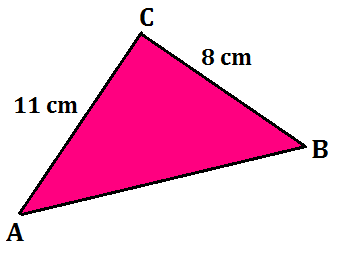So, 2s = 32, i.e., s = 16 cm,
s – a = (16 – 8) cm = 8 cm,
s – b = (16 – 11) cm = 5 cm,
s – c = (16 – 13) cm = 3 cm.

Therefore, the area of the triangle =
√16 x 8 x 5 x 3 = 8√30 cm²

Example 2: Find the area of a right-angled triangle with a base of 14 cm and a height of 5 cm.

Solution: Area of a right-angled triangle = 1/2 x b x h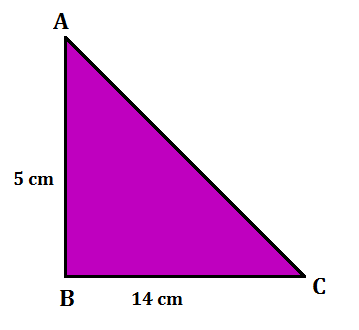Hence, the area of the right-angled triangle is

A= 1/2 x 14 x 5 = 35 cm²

Sharing is caring!

June Current Affairs PDF

×

Thank You, Your details have been submitted we will get back to you.Join India's largest learning destination

What You Will get ?

•Job Alerts
•Daily Quizzes
•Subject-Wise Quizzes
•Current Affairs
•Previous year question papers
•Doubt Solving session

ORJoin India's largest learning destination

What You Will get ?

•Job Alerts
•Daily Quizzes
•Subject-Wise Quizzes
•Current Affairs
•Previous year question papers
•Doubt Solving session

ORJoin India's largest learning destination

What You Will get ?

•Job Alerts
•Daily Quizzes
•Subject-Wise Quizzes
•Current Affairs
•Previous year question papers
•Doubt Solving session

Enter the email address associated with your account, and we'll email you an OTP to verify it's you.Join India's largest learning destination

What You Will get ?

•Job Alerts
•Daily Quizzes
•Subject-Wise Quizzes
•Current Affairs
•Previous year question papers
•Doubt Solving session

Enter OTP

Please enter the OTP sent to
/6

Did not recive OTP?

Resend in 60sJoin India's largest learning destination

What You Will get ?

•Job Alerts
•Daily Quizzes
•Subject-Wise Quizzes
•Current Affairs
•Previous year question papers
•Doubt Solving sessionJoin India's largest learning destination

What You Will get ?

•Job Alerts
•Daily Quizzes
•Subject-Wise Quizzes
•Current Affairs
•Previous year question papers
•Doubt Solving session

Almost there

+91Join India's largest learning destination

What You Will get ?

•Job Alerts
•Daily Quizzes
•Subject-Wise Quizzes
•Current Affairs
•Previous year question papers
•Doubt Solving session

Enter OTP

Please enter the OTP sent to Edit Number

Did not recive OTP?

Resend 60

By skipping this step you will not recieve any free content avalaible on adda247, also you will miss onto notification and job alerts

Are you sure you want to skip this step?

By skipping this step you will not recieve any free content avalaible on adda247, also you will miss onto notification and job alerts

Are you sure you want to skip this step?